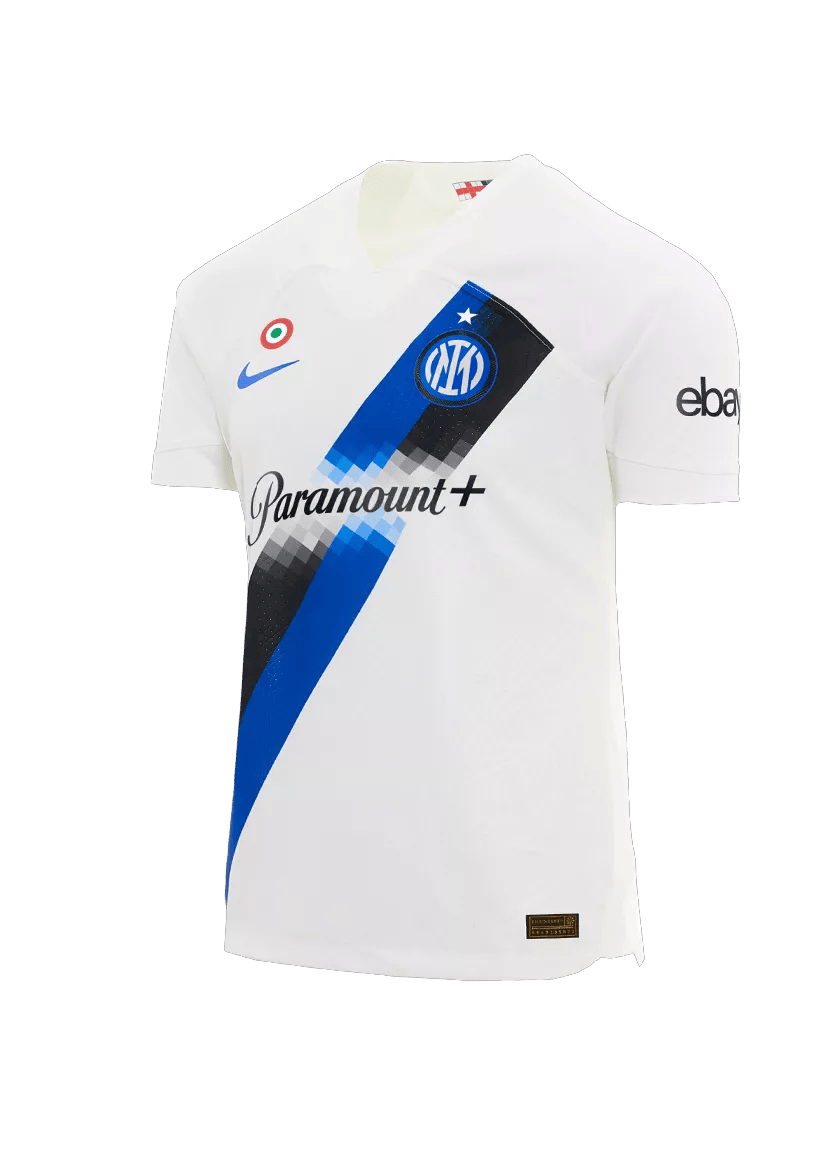# .css-adzqt3{display:inline-block;word-break:break-word;opacity:0;position:relative;-webkit-animation:animation-plwpox 0ms 0.4s forwards;animation:animation-plwpox 0ms 0.4s forwards;}.css-adzqt3::after{content:"";position:absolute;width:calc(100% + 14px);height:100%;top:0px;left:0px;background-color:var(--chakra-colors-light-white_100);-webkit-transform:scale3d(0, 1.05, 1);-moz-transform:scale3d(0, 1.05, 1);-ms-transform:scale3d(0, 1.05, 1);transform:scale3d(0, 1.05, 1);-webkit-animation:animation-f981je 250ms 0.15000000000000002s forwards step-end,animation-1ncn112 250ms 0.65s forwards step-end;animation:animation-f981je 250ms 0.15000000000000002s forwards step-end,animation-1ncn112 250ms 0.65s forwards step-end;}@-webkit-keyframes animation-1ncn112{0%{-webkit-transform:scale3d(0.75, 1.05, 1);-moz-transform:scale3d(0.75, 1.05, 1);-ms-transform:scale3d(0.75, 1.05, 1);transform:scale3d(0.75, 1.05, 1);transform-origin:top right;}44.4%{-webkit-transform:scale3d(0.25, 1.05, 1);-moz-transform:scale3d(0.25, 1.05, 1);-ms-transform:scale3d(0.25, 1.05, 1);transform:scale3d(0.25, 1.05, 1);transform-origin:top right;}100%{-webkit-transform:scale3d(0, 1.05, 1);-moz-transform:scale3d(0, 1.05, 1);-ms-transform:scale3d(0, 1.05, 1);transform:scale3d(0, 1.05, 1);transform-origin:top right;}}@keyframes animation-1ncn112{0%{-webkit-transform:scale3d(0.75, 1.05, 1);-moz-transform:scale3d(0.75, 1.05, 1);-ms-transform:scale3d(0.75, 1.05, 1);transform:scale3d(0.75, 1.05, 1);transform-origin:top right;}44.4%{-webkit-transform:scale3d(0.25, 1.05, 1);-moz-transform:scale3d(0.25, 1.05, 1);-ms-transform:scale3d(0.25, 1.05, 1);transform:scale3d(0.25, 1.05, 1);transform-origin:top right;}100%{-webkit-transform:scale3d(0, 1.05, 1);-moz-transform:scale3d(0, 1.05, 1);-ms-transform:scale3d(0, 1.05, 1);transform:scale3d(0, 1.05, 1);transform-origin:top right;}}@-webkit-keyframes animation-f981je{0%{-webkit-transform:scale3d(0, 1.05, 1);-moz-transform:scale3d(0, 1.05, 1);-ms-transform:scale3d(0, 1.05, 1);transform:scale3d(0, 1.05, 1);transform-origin:top left;}22.2%{-webkit-transform:scale3d(0.25, 1.05, 1);-moz-transform:scale3d(0.25, 1.05, 1);-ms-transform:scale3d(0.25, 1.05, 1);transform:scale3d(0.25, 1.05, 1);transform-origin:top left;}44.4%{-webkit-transform:scale3d(0.5, 1.05, 1);-moz-transform:scale3d(0.5, 1.05, 1);-ms-transform:scale3d(0.5, 1.05, 1);transform:scale3d(0.5, 1.05, 1);transform-origin:top left;}66.6%{-webkit-transform:scale3d(0.75, 1.05, 1);-moz-transform:scale3d(0.75, 1.05, 1);-ms-transform:scale3d(0.75, 1.05, 1);transform:scale3d(0.75, 1.05, 1);transform-origin:top left;}100%{-webkit-transform:scale3d(1, 1.05, 1);-moz-transform:scale3d(1, 1.05, 1);-ms-transform:scale3d(1, 1.05, 1);transform:scale3d(1, 1.05, 1);transform-origin:top left;}}@keyframes animation-f981je{0%{-webkit-transform:scale3d(0, 1.05, 1);-moz-transform:scale3d(0, 1.05, 1);-ms-transform:scale3d(0, 1.05, 1);transform:scale3d(0, 1.05, 1);transform-origin:top left;}22.2%{-webkit-transform:scale3d(0.25, 1.05, 1);-moz-transform:scale3d(0.25, 1.05, 1);-ms-transform:scale3d(0.25, 1.05, 1);transform:scale3d(0.25, 1.05, 1);transform-origin:top left;}44.4%{-webkit-transform:scale3d(0.5, 1.05, 1);-moz-transform:scale3d(0.5, 1.05, 1);-ms-transform:scale3d(0.5, 1.05, 1);transform:scale3d(0.5, 1.05, 1);transform-origin:top left;}66.6%{-webkit-transform:scale3d(0.75, 1.05, 1);-moz-transform:scale3d(0.75, 1.05, 1);-ms-transform:scale3d(0.75, 1.05, 1);transform:scale3d(0.75, 1.05, 1);transform-origin:top left;}100%{-webkit-transform:scale3d(1, 1.05, 1);-moz-transform:scale3d(1, 1.05, 1);-ms-transform:scale3d(1, 1.05, 1);transform:scale3d(1, 1.05, 1);transform-origin:top left;}}@-webkit-keyframes animation-plwpox{from{opacity:0;}to{opacity:1;}}@keyframes animation-plwpox{from{opacity:0;}to{opacity:1;}}NEVER.css-z4mzdx{display:inline-block;word-break:break-word;opacity:0;position:relative;-webkit-animation:animation-plwpox 0ms 0.65s forwards;animation:animation-plwpox 0ms 0.65s forwards;}.css-z4mzdx::after{content:"";position:absolute;width:calc(100% + 14px);height:100%;top:0px;left:0px;background-color:var(--chakra-colors-light-white_100);-webkit-transform:scale3d(0, 1.05, 1);-moz-transform:scale3d(0, 1.05, 1);-ms-transform:scale3d(0, 1.05, 1);transform:scale3d(0, 1.05, 1);-webkit-animation:animation-f981je 250ms 0.4s forwards step-end,animation-1ncn112 250ms 0.9s forwards step-end;animation:animation-f981je 250ms 0.4s forwards step-end,animation-1ncn112 250ms 0.9s forwards step-end;}@-webkit-keyframes animation-1ncn112{0%{-webkit-transform:scale3d(0.75, 1.05, 1);-moz-transform:scale3d(0.75, 1.05, 1);-ms-transform:scale3d(0.75, 1.05, 1);transform:scale3d(0.75, 1.05, 1);transform-origin:top right;}44.4%{-webkit-transform:scale3d(0.25, 1.05, 1);-moz-transform:scale3d(0.25, 1.05, 1);-ms-transform:scale3d(0.25, 1.05, 1);transform:scale3d(0.25, 1.05, 1);transform-origin:top right;}100%{-webkit-transform:scale3d(0, 1.05, 1);-moz-transform:scale3d(0, 1.05, 1);-ms-transform:scale3d(0, 1.05, 1);transform:scale3d(0, 1.05, 1);transform-origin:top right;}}@keyframes animation-1ncn112{0%{-webkit-transform:scale3d(0.75, 1.05, 1);-moz-transform:scale3d(0.75, 1.05, 1);-ms-transform:scale3d(0.75, 1.05, 1);transform:scale3d(0.75, 1.05, 1);transform-origin:top right;}44.4%{-webkit-transform:scale3d(0.25, 1.05, 1);-moz-transform:scale3d(0.25, 1.05, 1);-ms-transform:scale3d(0.25, 1.05, 1);transform:scale3d(0.25, 1.05, 1);transform-origin:top right;}100%{-webkit-transform:scale3d(0, 1.05, 1);-moz-transform:scale3d(0, 1.05, 1);-ms-transform:scale3d(0, 1.05, 1);transform:scale3d(0, 1.05, 1);transform-origin:top right;}}@-webkit-keyframes animation-f981je{0%{-webkit-transform:scale3d(0, 1.05, 1);-moz-transform:scale3d(0, 1.05, 1);-ms-transform:scale3d(0, 1.05, 1);transform:scale3d(0, 1.05, 1);transform-origin:top left;}22.2%{-webkit-transform:scale3d(0.25, 1.05, 1);-moz-transform:scale3d(0.25, 1.05, 1);-ms-transform:scale3d(0.25, 1.05, 1);transform:scale3d(0.25, 1.05, 1);transform-origin:top left;}44.4%{-webkit-transform:scale3d(0.5, 1.05, 1);-moz-transform:scale3d(0.5, 1.05, 1);-ms-transform:scale3d(0.5, 1.05, 1);transform:scale3d(0.5, 1.05, 1);transform-origin:top left;}66.6%{-webkit-transform:scale3d(0.75, 1.05, 1);-moz-transform:scale3d(0.75, 1.05, 1);-ms-transform:scale3d(0.75, 1.05, 1);transform:scale3d(0.75, 1.05, 1);transform-origin:top left;}100%{-webkit-transform:scale3d(1, 1.05, 1);-moz-transform:scale3d(1, 1.05, 1);-ms-transform:scale3d(1, 1.05, 1);transform:scale3d(1, 1.05, 1);transform-origin:top left;}}@keyframes animation-f981je{0%{-webkit-transform:scale3d(0, 1.05, 1);-moz-transform:scale3d(0, 1.05, 1);-ms-transform:scale3d(0, 1.05, 1);transform:scale3d(0, 1.05, 1);transform-origin:top left;}22.2%{-webkit-transform:scale3d(0.25, 1.05, 1);-moz-transform:scale3d(0.25, 1.05, 1);-ms-transform:scale3d(0.25, 1.05, 1);transform:scale3d(0.25, 1.05, 1);transform-origin:top left;}44.4%{-webkit-transform:scale3d(0.5, 1.05, 1);-moz-transform:scale3d(0.5, 1.05, 1);-ms-transform:scale3d(0.5, 1.05, 1);transform:scale3d(0.5, 1.05, 1);transform-origin:top left;}66.6%{-webkit-transform:scale3d(0.75, 1.05, 1);-moz-transform:scale3d(0.75, 1.05, 1);-ms-transform:scale3d(0.75, 1.05, 1);transform:scale3d(0.75, 1.05, 1);transform-origin:top left;}100%{-webkit-transform:scale3d(1, 1.05, 1);-moz-transform:scale3d(1, 1.05, 1);-ms-transform:scale3d(1, 1.05, 1);transform:scale3d(1, 1.05, 1);transform-origin:top left;}}@-webkit-keyframes animation-plwpox{from{opacity:0;}to{opacity:1;}}@keyframes animation-plwpox{from{opacity:0;}to{opacity:1;}}AWAY.css-1hstmlz{display:inline-block;word-break:break-word;opacity:0;position:relative;-webkit-animation:animation-plwpox 0ms 0.9s forwards;animation:animation-plwpox 0ms 0.9s forwards;}.css-1hstmlz::after{content:"";position:absolute;width:calc(100% + 14px);height:100%;top:0px;left:0px;background-color:var(--chakra-colors-light-white_100);-webkit-transform:scale3d(0, 1.05, 1);-moz-transform:scale3d(0, 1.05, 1);-ms-transform:scale3d(0, 1.05, 1);transform:scale3d(0, 1.05, 1);-webkit-animation:animation-f981je 250ms 0.65s forwards step-end,animation-1ncn112 250ms 1.15s forwards step-end;animation:animation-f981je 250ms 0.65s forwards step-end,animation-1ncn112 250ms 1.15s forwards step-end;}@-webkit-keyframes animation-1ncn112{0%{-webkit-transform:scale3d(0.75, 1.05, 1);-moz-transform:scale3d(0.75, 1.05, 1);-ms-transform:scale3d(0.75, 1.05, 1);transform:scale3d(0.75, 1.05, 1);transform-origin:top right;}44.4%{-webkit-transform:scale3d(0.25, 1.05, 1);-moz-transform:scale3d(0.25, 1.05, 1);-ms-transform:scale3d(0.25, 1.05, 1);transform:scale3d(0.25, 1.05, 1);transform-origin:top right;}100%{-webkit-transform:scale3d(0, 1.05, 1);-moz-transform:scale3d(0, 1.05, 1);-ms-transform:scale3d(0, 1.05, 1);transform:scale3d(0, 1.05, 1);transform-origin:top right;}}@keyframes animation-1ncn112{0%{-webkit-transform:scale3d(0.75, 1.05, 1);-moz-transform:scale3d(0.75, 1.05, 1);-ms-transform:scale3d(0.75, 1.05, 1);transform:scale3d(0.75, 1.05, 1);transform-origin:top right;}44.4%{-webkit-transform:scale3d(0.25, 1.05, 1);-moz-transform:scale3d(0.25, 1.05, 1);-ms-transform:scale3d(0.25, 1.05, 1);transform:scale3d(0.25, 1.05, 1);transform-origin:top right;}100%{-webkit-transform:scale3d(0, 1.05, 1);-moz-transform:scale3d(0, 1.05, 1);-ms-transform:scale3d(0, 1.05, 1);transform:scale3d(0, 1.05, 1);transform-origin:top right;}}@-webkit-keyframes animation-f981je{0%{-webkit-transform:scale3d(0, 1.05, 1);-moz-transform:scale3d(0, 1.05, 1);-ms-transform:scale3d(0, 1.05, 1);transform:scale3d(0, 1.05, 1);transform-origin:top left;}22.2%{-webkit-transform:scale3d(0.25, 1.05, 1);-moz-transform:scale3d(0.25, 1.05, 1);-ms-transform:scale3d(0.25, 1.05, 1);transform:scale3d(0.25, 1.05, 1);transform-origin:top left;}44.4%{-webkit-transform:scale3d(0.5, 1.05, 1);-moz-transform:scale3d(0.5, 1.05, 1);-ms-transform:scale3d(0.5, 1.05, 1);transform:scale3d(0.5, 1.05, 1);transform-origin:top left;}66.6%{-webkit-transform:scale3d(0.75, 1.05, 1);-moz-transform:scale3d(0.75, 1.05, 1);-ms-transform:scale3d(0.75, 1.05, 1);transform:scale3d(0.75, 1.05, 1);transform-origin:top left;}100%{-webkit-transform:scale3d(1, 1.05, 1);-moz-transform:scale3d(1, 1.05, 1);-ms-transform:scale3d(1, 1.05, 1);transform:scale3d(1, 1.05, 1);transform-origin:top left;}}@keyframes animation-f981je{0%{-webkit-transform:scale3d(0, 1.05, 1);-moz-transform:scale3d(0, 1.05, 1);-ms-transform:scale3d(0, 1.05, 1);transform:scale3d(0, 1.05, 1);transform-origin:top left;}22.2%{-webkit-transform:scale3d(0.25, 1.05, 1);-moz-transform:scale3d(0.25, 1.05, 1);-ms-transform:scale3d(0.25, 1.05, 1);transform:scale3d(0.25, 1.05, 1);transform-origin:top left;}44.4%{-webkit-transform:scale3d(0.5, 1.05, 1);-moz-transform:scale3d(0.5, 1.05, 1);-ms-transform:scale3d(0.5, 1.05, 1);transform:scale3d(0.5, 1.05, 1);transform-origin:top left;}66.6%{-webkit-transform:scale3d(0.75, 1.05, 1);-moz-transform:scale3d(0.75, 1.05, 1);-ms-transform:scale3d(0.75, 1.05, 1);transform:scale3d(0.75, 1.05, 1);transform-origin:top left;}100%{-webkit-transform:scale3d(1, 1.05, 1);-moz-transform:scale3d(1, 1.05, 1);-ms-transform:scale3d(1, 1.05, 1);transform:scale3d(1, 1.05, 1);transform-origin:top left;}}@-webkit-keyframes animation-plwpox{from{opacity:0;}to{opacity:1;}}@keyframes animation-plwpox{from{opacity:0;}to{opacity:1;}}-.css-nzcnf6{display:inline-block;word-break:break-word;opacity:0;position:relative;-webkit-animation:animation-plwpox 0ms 1.15s forwards;animation:animation-plwpox 0ms 1.15s forwards;}.css-nzcnf6::after{content:"";position:absolute;width:calc(100% + 14px);height:100%;top:0px;left:0px;background-color:var(--chakra-colors-light-white_100);-webkit-transform:scale3d(0, 1.05, 1);-moz-transform:scale3d(0, 1.05, 1);-ms-transform:scale3d(0, 1.05, 1);transform:scale3d(0, 1.05, 1);-webkit-animation:animation-f981je 250ms 0.9s forwards step-end,animation-1ncn112 250ms 1.4s forwards step-end;animation:animation-f981je 250ms 0.9s forwards step-end,animation-1ncn112 250ms 1.4s forwards step-end;}@-webkit-keyframes animation-1ncn112{0%{-webkit-transform:scale3d(0.75, 1.05, 1);-moz-transform:scale3d(0.75, 1.05, 1);-ms-transform:scale3d(0.75, 1.05, 1);transform:scale3d(0.75, 1.05, 1);transform-origin:top right;}44.4%{-webkit-transform:scale3d(0.25, 1.05, 1);-moz-transform:scale3d(0.25, 1.05, 1);-ms-transform:scale3d(0.25, 1.05, 1);transform:scale3d(0.25, 1.05, 1);transform-origin:top right;}100%{-webkit-transform:scale3d(0, 1.05, 1);-moz-transform:scale3d(0, 1.05, 1);-ms-transform:scale3d(0, 1.05, 1);transform:scale3d(0, 1.05, 1);transform-origin:top right;}}@keyframes animation-1ncn112{0%{-webkit-transform:scale3d(0.75, 1.05, 1);-moz-transform:scale3d(0.75, 1.05, 1);-ms-transform:scale3d(0.75, 1.05, 1);transform:scale3d(0.75, 1.05, 1);transform-origin:top right;}44.4%{-webkit-transform:scale3d(0.25, 1.05, 1);-moz-transform:scale3d(0.25, 1.05, 1);-ms-transform:scale3d(0.25, 1.05, 1);transform:scale3d(0.25, 1.05, 1);transform-origin:top right;}100%{-webkit-transform:scale3d(0, 1.05, 1);-moz-transform:scale3d(0, 1.05, 1);-ms-transform:scale3d(0, 1.05, 1);transform:scale3d(0, 1.05, 1);transform-origin:top right;}}@-webkit-keyframes animation-f981je{0%{-webkit-transform:scale3d(0, 1.05, 1);-moz-transform:scale3d(0, 1.05, 1);-ms-transform:scale3d(0, 1.05, 1);transform:scale3d(0, 1.05, 1);transform-origin:top left;}22.2%{-webkit-transform:scale3d(0.25, 1.05, 1);-moz-transform:scale3d(0.25, 1.05, 1);-ms-transform:scale3d(0.25, 1.05, 1);transform:scale3d(0.25, 1.05, 1);transform-origin:top left;}44.4%{-webkit-transform:scale3d(0.5, 1.05, 1);-moz-transform:scale3d(0.5, 1.05, 1);-ms-transform:scale3d(0.5, 1.05, 1);transform:scale3d(0.5, 1.05, 1);transform-origin:top left;}66.6%{-webkit-transform:scale3d(0.75, 1.05, 1);-moz-transform:scale3d(0.75, 1.05, 1);-ms-transform:scale3d(0.75, 1.05, 1);transform:scale3d(0.75, 1.05, 1);transform-origin:top left;}100%{-webkit-transform:scale3d(1, 1.05, 1);-moz-transform:scale3d(1, 1.05, 1);-ms-transform:scale3d(1, 1.05, 1);transform:scale3d(1, 1.05, 1);transform-origin:top left;}}@keyframes animation-f981je{0%{-webkit-transform:scale3d(0, 1.05, 1);-moz-transform:scale3d(0, 1.05, 1);-ms-transform:scale3d(0, 1.05, 1);transform:scale3d(0, 1.05, 1);transform-origin:top left;}22.2%{-webkit-transform:scale3d(0.25, 1.05, 1);-moz-transform:scale3d(0.25, 1.05, 1);-ms-transform:scale3d(0.25, 1.05, 1);transform:scale3d(0.25, 1.05, 1);transform-origin:top left;}44.4%{-webkit-transform:scale3d(0.5, 1.05, 1);-moz-transform:scale3d(0.5, 1.05, 1);-ms-transform:scale3d(0.5, 1.05, 1);transform:scale3d(0.5, 1.05, 1);transform-origin:top left;}66.6%{-webkit-transform:scale3d(0.75, 1.05, 1);-moz-transform:scale3d(0.75, 1.05, 1);-ms-transform:scale3d(0.75, 1.05, 1);transform:scale3d(0.75, 1.05, 1);transform-origin:top left;}100%{-webkit-transform:scale3d(1, 1.05, 1);-moz-transform:scale3d(1, 1.05, 1);-ms-transform:scale3d(1, 1.05, 1);transform:scale3d(1, 1.05, 1);transform-origin:top left;}}@-webkit-keyframes animation-plwpox{from{opacity:0;}to{opacity:1;}}@keyframes animation-plwpox{from{opacity:0;}to{opacity:1;}}国际米兰客场球衣重塑标志性的设计，致敬俱乐部国际灵魂

team

Jul 31st 2023

## 国际米兰发布新赛季客场球衣。

​“Never Away”，我们总有家的感觉，无论距离有多远。国际米兰的家即世界，无论身在何处，你都会找到黑与蓝相遇之地，因为我们生而国际。

INTER ONLINE STORE

NEVER AWAY - Inter Away Kit 2023/2024## 相关新闻team

Yesterday

• 俱乐部

• Inter Store